# tsfresh.feature_extraction package¶

## tsfresh.feature_extraction.extraction module¶

This module contains the main function to interact with tsfresh: extract features

tsfresh.feature_extraction.extraction.extract_features(timeseries_container, feature_extraction_settings=None, column_id=None, column_sort=None, column_kind=None, column_value=None)[source]

Extract features from

or

In both cases a pandas.DataFrame with the calculated features will be returned.

For a list of all the calculated time series features, please see the FeatureExtractionSettings class, which is used to control which features with which parameters are calculated.

For a detailed explanation of the different parameters and data formats please see Data Formats.

Examples

>>> from tsfresh.examples import load_robot_execution_failures
>>> from tsfresh import extract_features
>>> X = extract_features(df, column_id='id', column_sort='time')


which would give the same results as described above. In this case, the column_kind is not allowed. Except that, the same rules for leaving out the columns apply as above.

Parameters: timeseries_container (pandas.DataFrame or dict) – The pandas.DataFrame with the time series to compute the features for, or a dictionary of pandas.DataFrames. column_id (str) – The name of the id column to group by. column_sort (str) – The name of the sort column. column_kind (str) – The name of the column keeping record on the kind of the value. column_value (str) – The name for the column keeping the value itself. feature_extraction_settings (tsfresh.feature_extraction.settings.FeatureExtractionSettings) – settings object that controls which features are calculated The (maybe imputed) DataFrame with the extracted features. pandas.DataFrame

## tsfresh.feature_extraction.feature_calculators module¶

This module contains the feature calculators that take time series as input and calculate the values of the feature. There are three types of features:

1. aggregate features without parameter
2. aggregate features with parameter
3. apply features with parameters

While type 1 and 2 are designed to be used with pandas aggregate, they will only return one singular feature. To not unnecessarily redo auxiliary calculations, in type 3 a group of features is calculated at the same time. They can be used with pandas apply.

tsfresh.feature_extraction.feature_calculators.abs_energy(x, *arg, **args)[source]

Returns the absolute energy of the time series which is the sum over the squared values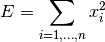Parameters: x (pandas.Series) – the time series to calculate the feature of the value of this feature float

This function is of type: aggregate

tsfresh.feature_extraction.feature_calculators.absolute_sum_of_changes(x, *arg, **args)[source]

Returns the sum over the absolute value of consecutive changes in the series x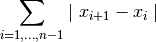Parameters: x (pandas.Series) – the time series to calculate the feature of the value of this feature float

This function is of type: aggregate

tsfresh.feature_extraction.feature_calculators.approximate_entropy(x, m, r)[source]

Implements a vectorized Approximate entropy algorithm.

For short time-series this method is highly dependent on the parameters, but should be stable for N > 2000, see:

Yentes et al. (2012) - The Appropriate Use of Approximate Entropy and Sample Entropy with Short Data Sets

Other shortcomings and alternatives discussed in:

Richman & Moorman (2000) - Physiological time-series analysis using approximate entropy and sample entropy
Parameters: x (pandas.Series) – the time series to calculate the feature of m (int) – Length of compared run of data r (float) – Filtering level, must be positive Approximate entropy float

This function is of type: aggregate_with_parameters

tsfresh.feature_extraction.feature_calculators.ar_coefficient(x, *arg, **args)[source]

This feature calculator fit the unconditional maximum likelihood of an autoregressive AR(k) process. The k parameter is the maximum lag of the process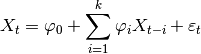For the configurations from param which should contain the maxlag “k” and such an AR process is calculated. Then the coefficients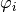whose indexcontained from “coeff” are returned.

Parameters: x (pandas.Series) – the time series to calculate the feature of c (str) – the time series name param (list) – contains dictionaries {“coeff”: x, “k”: y} with x,y int the different feature values pandas.Series

This function is of type: apply

tsfresh.feature_extraction.feature_calculators.augmented_dickey_fuller(x, *arg, **args)[source]

The Augmented Dickey-Fuller is a hypothesis test which checks whether a unit root is present in a time series sample. This feature calculator returns the value of the respective test statistic.

See the statsmodels implementation for references and more details.

Parameters: x (pandas.Series) – the time series to calculate the feature of the value of this feature float

This function is of type: aggregate

tsfresh.feature_extraction.feature_calculators.autocorrelation(x, *arg, **args)[source]

Calculates the lag autocorrelation of a lag value of lag.

Parameters: x (pandas.Series) – the time series to calculate the feature of lag (int) – the lag the value of this feature float

This function is of type: aggregate_with_parameters

tsfresh.feature_extraction.feature_calculators.binned_entropy(x, *arg, **args)[source]

First bins the values of x into max_bins equidistant bins. Then calculates the value of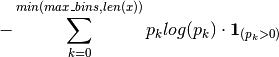where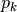is the percentage of samples in bin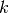.

Parameters: x (pandas.Series) – the time series to calculate the feature of max_bins (int) – the maximal number of bins the value of this feature float

This function is of type: aggregate_with_parameters

tsfresh.feature_extraction.feature_calculators.count_above_mean(x, *arg, **args)[source]

Returns the number of values in x that are higher than the mean of x

Parameters: x (pandas.Series) – the time series to calculate the feature of the value of this feature float

This function is of type: aggregate

tsfresh.feature_extraction.feature_calculators.count_below_mean(x, *arg, **args)[source]

Returns the number of values in x that are lower than the mean of x

Parameters: x (pandas.Series) – the time series to calculate the feature of the value of this feature float

This function is of type: aggregate

tsfresh.feature_extraction.feature_calculators.cwt_coefficients(x, *arg, **args)[source]

Calculates a Continuous wavelet transform for the Ricker wavelet, also known as the “Mexican hat wavelet” which is defined by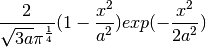where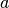is the width parameter of the wavelet function.

This feature calculator takes three different parameter: widths, coeff and w. The feature calculater takes all the different widths arrays and then calculates the cwt one time for each different width array. Then the values for the different coefficient for coeff and width w are returned. (For each dic in param one feature is returned)

Parameters: x (pandas.Series) – the time series to calculate the feature of c (str) – the time series name param (list) – contains dictionaries {“widths”:x, “coeff”: y, “w”: z} with x array of int and y,z int the different feature values pandas.Series

This function is of type: apply

tsfresh.feature_extraction.feature_calculators.fft_coefficient(x, *arg, **args)[source]

Calculates the fourier coefficients of the one-dimensional discrete Fourier Transform for real input by fast fourier transformation algorithm

Parameters: x (pandas.Series) – the time series to calculate the feature of c (str) – the time series name param (list) – contains dictionaries {“coeff”: x} with x int and x >= 0 the different feature values pandas.Series

This function is of type: apply

tsfresh.feature_extraction.feature_calculators.first_location_of_maximum(x, *arg, **args)[source]

Returns the first location of the maximum value of x. The position is calculated relatively to the length of x.

Parameters: x (pandas.Series) – the time series to calculate the feature of the value of this feature float

This function is of type: aggregate

tsfresh.feature_extraction.feature_calculators.first_location_of_minimum(x, *arg, **args)[source]

Returns the first location of the minimal value of x. The position is calculated relatively to the length of x.

Parameters: x (pandas.Series) – the time series to calculate the feature of the value of this feature float

This function is of type: aggregate

tsfresh.feature_extraction.feature_calculators.has_duplicate(x, *arg, **args)[source]

Checks if any value in x occurs more than once

Parameters: x (pandas.Series) – the time series to calculate the feature of the value of this feature bool

This function is of type: aggregate

tsfresh.feature_extraction.feature_calculators.has_duplicate_max(x, *arg, **args)[source]

Checks if the maximum value of x is observed more than once

Parameters: x (pandas.Series) – the time series to calculate the feature of the value of this feature bool

This function is of type: aggregate

tsfresh.feature_extraction.feature_calculators.has_duplicate_min(x, *arg, **args)[source]

Checks if the minimal value of x is observed more than once

Parameters: x (pandas.Series) – the time series to calculate the feature of the value of this feature bool

This function is of type: aggregate

tsfresh.feature_extraction.feature_calculators.index_mass_quantile(x, *arg, **args)[source]

Those apply features calculate the relative index i where q% of the mass of the time series x lie left of i. For example for q = 50% this feature calculator will return the mass center of the time series

Parameters: x (pandas.Series) – the time series to calculate the feature of c (str) – the time series name param (list) – contains dictionaries {“q”: x} with x float the different feature values pandas.Series

This function is of type: apply

tsfresh.feature_extraction.feature_calculators.kurtosis(x, *arg, **args)[source]

Returns the kurtosis of x (calculated with the adjusted Fisher-Pearson standardized moment coefficient G2).

Parameters: x (pandas.Series) – the time series to calculate the feature of the value of this feature float

This function is of type: aggregate

tsfresh.feature_extraction.feature_calculators.large_number_of_peaks(x, *arg, **args)[source]

Checks if the number of peaks is higher than n.

Parameters: x (pandas.Series) – the time series to calculate the feature of n (int) – the number of peaks to compare the value of this feature bool

This function is of type: aggregate_with_parameters

tsfresh.feature_extraction.feature_calculators.large_standard_deviation(x, *arg, **args)[source]

Boolean variable denoting if the variance of x is higher than half of the range, calculated as the half the difference between max and min of x. Hence it checks if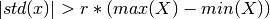According to a rule of the thumb, the standard deviation should be a forth of the range of the values.

Parameters: x (pandas.Series) – the time series to calculate the feature of r (float) – the percentage of the range to compare with the value of this feature bool

This function is of type: aggregate_with_parameters

tsfresh.feature_extraction.feature_calculators.last_location_of_maximum(x, *arg, **args)[source]

Returns the relative last location of the maximum value of x. The position is calculated relatively to the length of x.

Parameters: x (pandas.Series) – the time series to calculate the feature of the value of this feature float

This function is of type: aggregate

tsfresh.feature_extraction.feature_calculators.last_location_of_minimum(x, *arg, **args)[source]

Returns the last location of the minimal value of x. The position is calculated relatively to the length of x.

Parameters: x (pandas.Series) – the time series to calculate the feature of the value of this feature float

This function is of type: aggregate

tsfresh.feature_extraction.feature_calculators.length(x, *arg, **args)[source]

Returns the length of x

Parameters: x (pandas.Series) – the time series to calculate the feature of the value of this feature int

This function is of type: aggregate

tsfresh.feature_extraction.feature_calculators.longest_strike_above_mean(x, *arg, **args)[source]

Returns the length of the longest consecutive subsequence that in x that is bigger than the mean of x

Parameters: x (pandas.Series) – the time series to calculate the feature of the value of this feature float

This function is of type: aggregate

tsfresh.feature_extraction.feature_calculators.longest_strike_below_mean(x, *arg, **args)[source]

Returns the length of the longest consecutive subsequence that in x that is smaller than the mean of x

Parameters: x (pandas.Series) – the time series to calculate the feature of the value of this feature float

This function is of type: aggregate

tsfresh.feature_extraction.feature_calculators.maximum(x)[source]

Calculates the highest value of the time series x.

Parameters: x (pandas.Series) – the time series to calculate the feature of the value of this feature float

This function is of type: aggregate

tsfresh.feature_extraction.feature_calculators.mean(x)[source]

Returns the mean of x

Parameters: x (pandas.Series) – the time series to calculate the feature of the value of this feature float

This function is of type: aggregate

tsfresh.feature_extraction.feature_calculators.mean_abs_change(x, *arg, **args)[source]

Returns the mean over the absolute differences between subsequent time series values which is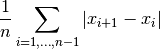Parameters: x (pandas.Series) – the time series to calculate the feature of the value of this feature float

This function is of type: aggregate

tsfresh.feature_extraction.feature_calculators.mean_abs_change_quantiles(x, *arg, **args)[source]

First fixes a corridor given by the quantiles ql and qh of the distribution of x. Then calculates the average absolute value of consecutive changes of the series x inside this corridor. Think about selecting a corridor on the y-Axis and only calculating the mean of the absolute change of the time series inside this corridor.

Parameters: x (pandas.Series) – the time series to calculate the feature of ql (float) – the lower quantile of the corridor qh (float) – the higher quantile of the corridor the value of this feature float

This function is of type: aggregate_with_parameters

tsfresh.feature_extraction.feature_calculators.mean_autocorrelation(x, *arg, **args)[source]

Calculates the average autocorrelation (Compare to http://en.wikipedia.org/wiki/Autocorrelation#Estimation), taken over different all possible lags (1 to length of x)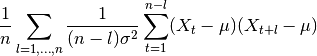where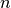is the length of the time series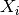,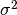its variance and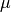its mean.

Parameters: x (pandas.Series) – the time series to calculate the feature of the value of this feature float

This function is of type: aggregate

tsfresh.feature_extraction.feature_calculators.mean_change(x, *arg, **args)[source]

Returns the mean over the absolute differences between subsequent time series values which is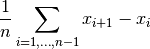Parameters: x (pandas.Series) – the time series to calculate the feature of the value of this feature float

This function is of type: aggregate

tsfresh.feature_extraction.feature_calculators.mean_second_derivate_central(x, *arg, **args)[source]

Returns the mean value of an central approximation of the second derivate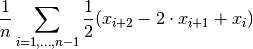Parameters: x (pandas.Series) – the time series to calculate the feature of the value of this feature float

This function is of type: aggregate

tsfresh.feature_extraction.feature_calculators.median(x)[source]

Returns the median of x

Parameters: x (pandas.Series) – the time series to calculate the feature of the value of this feature float

This function is of type: aggregate

tsfresh.feature_extraction.feature_calculators.minimum(x)[source]

Calculates the lowest value of the time series x.

Parameters: x (pandas.Series) – the time series to calculate the feature of the value of this feature float

This function is of type: aggregate

tsfresh.feature_extraction.feature_calculators.not_apply_to_raw_numbers(func)[source]

This decorator makes sure that the function func is only called on objects that are not numbers.Number

Parameters: func – the method that should only be executed on objects which are not a numbers.Number the decorated version of func which returns 0 if the first argument x is a numbers.Number. For every other x the output of func is returned
tsfresh.feature_extraction.feature_calculators.number_cwt_peaks(x, *arg, **args)[source]

This feature calculator searches for different peaks in x. To do so, x is smoothed by a ricker wavelet and for widths ranging from 1 to n. This feature calculator returns the number of peaks that occur at enough width scales and with sufficiently high Signal-to-Noise-Ratio (SNR)

Parameters: x (pandas.Series) – the time series to calculate the feature of n (int) – maximum width to consider the value of this feature int

This function is of type: aggregate_with_parameters

tsfresh.feature_extraction.feature_calculators.number_peaks(x, *arg, **args)[source]

Calculates the number of peaks of at least support n in the time series x. A peak of support n is defined as a subsequence of x where a value occurs, which is bigger than its n neighbours to the left and to the right.

Hence in the sequence

>>> x = [3, 0, 0, 4, 0, 0, 13]


4 is a peak of support 1 and 2 because in the subsequences

>>> [0, 4, 0]
>>> [0, 0, 4, 0, 0]


4 is still the highest value. Here, 4 is not a peak of support 3 because 13 is the 3th neighbour to the right of 4 and its bigger than 4.

Parameters: x (pandas.Series) – the time series to calculate the feature of n (int) – the support of the peak the value of this feature float

This function is of type: aggregate_with_parameters

tsfresh.feature_extraction.feature_calculators.percentage_of_reoccurring_datapoints_to_all_datapoints(x, *arg, **args)[source]

Returns the percentage of unique values, that are present in the time series more than once.

len(different values occurring more than once) / len(different values)

This means the percentage is normalized to the number of unique values, in contrast to the percentage_of_reoccurring_values_to_all_values.

Parameters: x (pandas.Series) – the time series to calculate the feature of the value of this feature float

This function is of type: aggregate

tsfresh.feature_extraction.feature_calculators.percentage_of_reoccurring_values_to_all_values(x, *arg, **args)[source]

Returns the ratio of unique values, that are present in the time series more than once.

# of data points occurring more than once / # of all data points

This means the ratio is normalized to the number of data points in the time series, in contrast to the percentage_of_reoccurring_datapoints_to_all_datapoints.

Parameters: x (pandas.Series) – the time series to calculate the feature of the value of this feature float

This function is of type: aggregate

tsfresh.feature_extraction.feature_calculators.quantile(x, *arg, **args)[source]

Calculates the q quantile of x. This is the value of x such that q% of the ordere values from x are lower than.

Parameters: x (pandas.Series) – the time series to calculate the feature of q (float) – the quantile to calculate the value of this feature float

This function is of type: aggregate_with_parameters

tsfresh.feature_extraction.feature_calculators.range_count(x, min, max)[source]

Count observed values within the interval [min, max).

Parameters: x (pandas.Series) – the time series to calculate the feature of min (int or float) – the inclusive lower bound of the range max (int or float) – the exclusive upper bound of the range the count of values within the range int

This function is of type: aggregate_with_parameters

tsfresh.feature_extraction.feature_calculators.ratio_value_number_to_time_series_length(x, *arg, **args)[source]

Returns a factor which is 1 if all values in the time series occur only once, and below one if this is not the case. In principle, it just returns

# unique values / # values
Parameters: x (pandas.Series) – the time series to calculate the feature of the value of this feature float

This function is of type: aggregate

tsfresh.feature_extraction.feature_calculators.sample_entropy(x)[source]

Calculate and return sample entropy of x. References: ———-  http://en.wikipedia.org/wiki/Sample_Entropy  https://www.ncbi.nlm.nih.gov/pubmed/10843903?dopt=Abstract

Parameters: x (pandas.Series) – the time series to calculate the feature of tolerance (float) – normalization factor; equivalent to the common practice of expressing the tolerance as r times the standard deviation the value of this feature float

This function is of type: aggregate

tsfresh.feature_extraction.feature_calculators.set_property(key, value)[source]

This method returns a decorator that sets the property key of the function to value

tsfresh.feature_extraction.feature_calculators.skewness(x, *arg, **args)[source]

Returns the sample skewness of x (calculated with the adjusted Fisher-Pearson standardized moment coefficient G1).

Parameters: x (pandas.Series) – the time series to calculate the feature of the value of this feature float

This function is of type: aggregate

tsfresh.feature_extraction.feature_calculators.spkt_welch_density(x, *arg, **args)[source]

This feature calculator estimates the cross power spectral density of the time series x at different frequencies. To do so, first the time series is shifted from the time domain to the frequency domain.

The feature calculators returns the power spectrum of the different frequencies.

Parameters: x (pandas.Series) – the time series to calculate the feature of c (str) – the time series name param (list) – contains dictionaries {“coeff”: x} with x int the different feature values pandas.Series

This function is of type: apply

tsfresh.feature_extraction.feature_calculators.standard_deviation(x, *arg, **args)[source]

Returns the standard deviation of x

Parameters: x (pandas.Series) – the time series to calculate the feature of the value of this feature float

This function is of type: aggregate

tsfresh.feature_extraction.feature_calculators.sum_of_reoccurring_values(x, *arg, **args)[source]

Returns the sum of all values, that are present in the time series more than once.

Parameters: x (pandas.Series) – the time series to calculate the feature of the value of this feature float

This function is of type: aggregate

tsfresh.feature_extraction.feature_calculators.sum_values(x)[source]

Calculates the sum over the time series values

Parameters: x (pandas.Series) – the time series to calculate the feature of the value of this feature bool

This function is of type: aggregate

tsfresh.feature_extraction.feature_calculators.symmetry_looking(x, *arg, **args)[source]

Boolean variable denoting if the distribution of x looks symmetric. This is the case if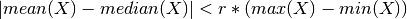Parameters: x (pandas.Series) – the time series to calculate the feature of r (float) – the percentage of the range to compare with the value of this feature bool

This function is of type: aggregate_with_parameters

tsfresh.feature_extraction.feature_calculators.time_reversal_asymmetry_statistic(x, *arg, **args)[source]

This function calculates the value of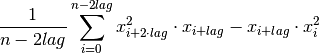which is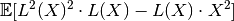where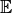is the mean and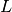is the lag operator. It was proposed as a proposed in  as a promising feature to extract from time series.

References

  Fulcher, B.D., Jones, N.S. (2014). Highly comparative feature-based time-series classification. Knowledge and Data Engineering, IEEE Transactions on 26, 3026–3037.
Parameters: x (pandas.Series) – the time series to calculate the feature of lag (int) – the lag that should be used in the calculation of the feature the value of this feature float

This function is of type: aggregate_with_parameters

tsfresh.feature_extraction.feature_calculators.value_count(x, value)[source]

Count occurrences of value in time series x.

Parameters: x (pandas.Series) – the time series to calculate the feature of value (int or float) – the value to be counted the count int

This function is of type: aggregate_with_parameters

tsfresh.feature_extraction.feature_calculators.variance(x, *arg, **args)[source]

Returns the variance of x

Parameters: x (pandas.Series) – the time series to calculate the feature of the value of this feature float

This function is of type: aggregate

tsfresh.feature_extraction.feature_calculators.variance_larger_than_standard_deviation(x, *arg, **args)[source]

Boolean variable denoting if the variance of x is greater than its standard deviation. Is equal to variance of x being larger than 1.

Parameters: x (pandas.Series) – the time series to calculate the feature of the value of this feature bool

This function is of type: aggregate

## tsfresh.feature_extraction.settings module¶

This file contains all settings of the tsfresh. For the naming of the features, see Feature naming.

class tsfresh.feature_extraction.settings.FeatureExtractionSettings(calculate_all_features=True)[source]

Bases: future.types.newobject.newobject

This class defines the behaviour of feature extraction, in particular which feature and parameter combinations are calculated. If you do not specify any user settings, all features will be extracted with default arguments defined in this class.

In general, we consider three types of time series features:

1. aggregate features without parameter that emit exactly one feature per function calculator
2. aggregate features with parameter that emit exactly one feature per function calculator
3. apply features with parameters that emit several features per function calculator (usually one feature per parameter value)

These three types are stored in different dictionaries. For the feature types with parameters there is also a dictionaries containing the parameters.

It is possible to obtain a FeatureExtractionSettings object from a feature matrix, see func:~tsfresh.feature_extraction.settings.FeatureExtractionSettings.from_columns. This is useful to reproduce the features of a train set for a test set.

To set user defined settings, do something like

>>> from tsfresh.feature_extraction import FeatureExtractionSettings
>>> settings = FeatureExtractionSettings()
>>> # Calculate all features except length
>>> settings.do_not_calculate("length")
>>> from tsfresh.feature_extraction import extract_features
>>> extract_features(df, feature_extraction_settings=settings)


Mostly, the settings in this class are for enabling/disabling the extraction of certain features, which can be important to save time during feature extraction. Additionally, some of the features have parameters which can be controlled here.

If the calculation of a feature failed (for whatever reason), the results can be NaN. The IMPUTE flag defaults to None and can be set to one of the impute functions in dataframe_functions.

do_not_calculate(kind, identifier)[source]

Delete the all features of type identifier for time series of type kind.

Parameters: kind (basestring) – the type of the time series identifier (basestring) – the name of the feature The setting object itself FeatureExtractionSettings
static from_columns(columns)[source]

Creates a FeatureExtractionSettings object set to extract only the features contained in the list columns. to do so, for every feature name in columns this method

1. split the column name into col, feature, params part
2. decide which feature we are dealing with (aggregate with/without params or apply)
3. add it to the new name_to_function dict
4. set up the params

Set the feature and params dictionaries in the settings object, then return it.

Parameters: columns (list of str) – containing the feature names The changed settings object FeatureExtractionSettings
get_aggregate_functions(kind)[source]

For the tyme series Returns a dictionary with the column name mapped to the feature calculators that are specified in the FeatureExtractionSettings object. This dictionary can be used in a pandas group by command to extract the all aggregate features at the same time.

Parameters: kind (basestring) – the type of the time series mapping of column name to function calculator dict
get_apply_functions(column_prefix)[source]

Convenience function to return a list with all the functions to apply on a data frame and extract features. Only adds those functions to the dictionary, that are enabled in the settings.

Parameters: column_prefix (basestring) – the prefix all column names. all functions to use for feature extraction list
static get_config_from_string(parts)[source]

Helper function to extract the configuration of a certain function from the column name. The column name parts (split by “__”) should be passed to this function. It will skip the kind name and the function name and only use the parameter parts. These parts will be split up on “_” into the parameter name and the parameter value. This value is transformed into a python object (for example is “(1, 2, 3)” transformed into a tuple consisting of the ints 1, 2 and 3).

Parameters: parts (list) – The column name split up on “__” a dictionary with all parameters, which are encoded in the column name. dict
set_default_parameters(kind)[source]

Setup the feature calculations for kind as defined in self.name_to_param

Parameters: kind – str, the type of the time series
class tsfresh.feature_extraction.settings.MinimalFeatureExtractionSettings[source]

This class is a parent class of the FeatureExtractionSettings class and has the same functionality as its base class. The only difference is, that most of the feature calculators are disabled and only a small subset of calculators will be calculated at all.

Use this class for quick tests of your setup before calculating all features which could take some time depending of your data set size.

You should use this object when calling the extract function, like so:

>>> from tsfresh.feature_extraction import extract_features, MinimalFeatureExtractionSettings
>>> extract_features(df, feature_extraction_settings=MinimalFeatureExtractionSettings)


## Module contents¶

The tsfresh.feature_extraction module contains methods to extract the features from the time series